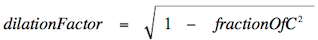### CSC 533: Organization of Programming Languages Spring 2016 HW5: Scheme Programming I

For this assignment, you are to define the following Scheme functions. For simplicity, place all of your function definitions in a single file named hw5.ss. Be sure to comment each function as to its behavior.

1. Define a function named windChill that takes two inputs, a temperature (in degrees Fahrenheit) and a wind speed (in miles per hour), and returns the corresponding wind chill factor. The formula for computing the wind chill is:

 windChill = { temperature if windSpeed <= 3 35.74 + 0.6215*temperature + (0.4275*temperature-35.75)*windSpeed0.16 otherwise
2. One of the consequences of Einstein's Theory of Relativity is the phenomenon of time dilation. As an observer increases in velocity, perceived time actually slows for that observer (relative to an outside observer). For everyday velocities, e.g., riding in a car or airplane, the effect is negligible. However, if spacecraft are eventually invented whose velocities approach the speed of light, the difference in relative time would be dramatic. For example, Betelguese is 309 light years from earth. Traveling at 0.5 of light speed, it would take 618 years for a ship to reach Betelguese (as observed from earth), but it would only seem like 267.6 years on board the ship. The factor by which relative time is reduced is defined by the following formula:Define a function named timeTravel that takes two inputs, a distance (in light years) and a velocity (a fraction of light speed). The function should return a pair of numbers, representing the absolute time (as observed from earth) and relative time (as observed on the ship) it would take to reach that distance at the given speed. For example, (timeTravel 309 0.5) should evaluate to (618.0 267.60184976939155).

3. Define functions for converting roman numerals to numbers and back. One function, roman->num will have one input, a list of characters representing a roman numeral. It should return the number value represented by that roman numeral. For example, (roman->num '(X V I)) should evaluate to 16. Conversely, the function num->roman will have an integer as input, and should return the list of characters representing the appropriate roman numeral. The following is a list of the roman letters and numbers they represent: M = 1000, D = 500, C = 100, L = 50, X = 10, V = 5, I = 1.

You may assume the ancient roman style of writing letters, where 4 is represented IIII and 90 is represented LXXXX. A harder problem, which you may attempt if you like, is to use the modern roman style where 4 is IV and 90 is XC.

4. Define a function named numCount1 that takes one input, a list containing arbitrary values, and returns a count of how many list elements are numbers. For example, (numCount1 '(z 4 (a 3) 2 foo)) should evaluate to 2.

5. Define a more general function named numCount2 that takes one input, a list containing arbitrary values, and returns a count of how many numbers appear anywhere in the list (including in nested sublists). For example, (numCount2 '(z 4 (a 3) 2 foo)) should evaluate to 3.

6. The `randomWalk` function below simulates a random 1-dimensional walk. Initially, the walker is assumed to be at position 0. Depending on the flip of a coin, the walker either moves to the right (in the positive direction) or to the left (in the negative direction). The simulation ends when the walker reaches the specified goal distance, in either direction. (define (coinFlip) (if (< (random) 0.5) 'heads 'tails)) (define (randomWalk goalDist) (define (randomWalk-help position) (begin (display "position: ") (display position) (newline) (cond ((= (abs position) goalDist) #t) ((equal? (coinFlip) 'heads) (randomWalk-help (add1 position))) (else (randomWalk-help (sub1 position)))))) (randomWalk-help 0))

As is, this function displays each step in the walk and returns #t when the walk ends. Modify the function so that it keeps track of the number of steps in the walk and returns this value instead. Your modification should only utilize tail-recursion.

7. Write a function named `head%` that simulates a specified number of coin flips and returns the percentage of those flips that were heads. For example, the call `(head% 1000)` would simulate 1,000 flips and return the percentage of heads, e.g., 50.8. Since the desired number of flips could be extremely large, your function should only utilize tail-recursion.

8. An interesting unsolved problem in mathematics concerns what is called the hailstone sequence of integers. This sequence is defined as follows: start with any integer. If that number is odd, then multiply it by 3 and add 1. If it is even, then divide it by 2. Now, repeat. For example, if we start with the number 5, we get the following sequence: 5, 16, 8, 4, 2, 1, 4, 2, 1, 4, 2, 1, . . . Here, the subsequence 4, 2, 1 is reached which produces a loop. It was conjectured (by mathematician Lothar Collatz) that no matter what number you start with, you will always end up stuck in the 4, 2, 1 loop. It has, in fact, been shown to hold for all starting values less than 5 x 260 ≈ 5.764 x 1018. However, it still has not been proven for all numbers.

Define a function named hailstone which has one input, the starting number, and which displays the hailstone sequence starting with that number and ending with 1. The function should return the length of the sequence. For example, (hailstone 1) should display the lone number 1 and evaluate to 1, while (hailstone 5) should display 5 16 8 4 2 1 and evaluate to 6. Your function should only utilize tail-recursion.